Torch-Code

valse2017/ppt

## 1. Introduction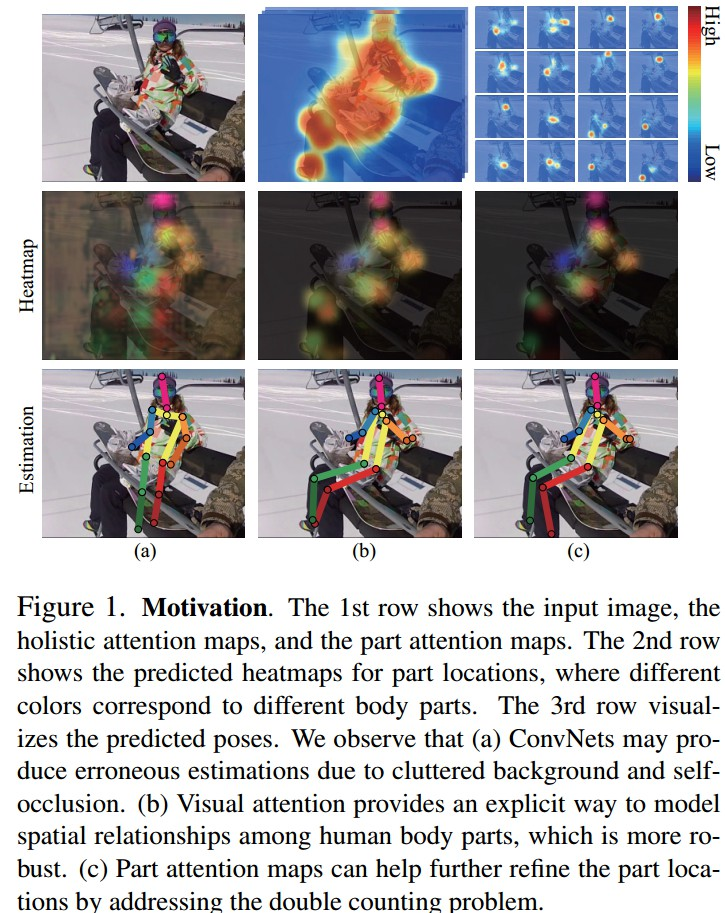Figure1. 第一行分别是，输入图片、整体注意力图、部分注意力图.

(a)由于背景复杂和自遮挡问题，ConvNets可能得到错误的估计结果.

(b)视觉注意力图对人体关节点的空间关系进行建模，鲁棒性好.

(c)关节点注意力图解决重复计算问题(double counting problem)，进一步提高关节点估计结果.

of interest)，采用注意力模型生成注意力图，只依赖图像特征，提供了更有效的方式来关注不同形状的目标区域.

## 2. 方法

Multi-Context Attention:
• Multi-Semantic Attention
• Multi-Resolution Attention
• Hierarchical (holistic/part) Attention
• Tailored Attention Scheme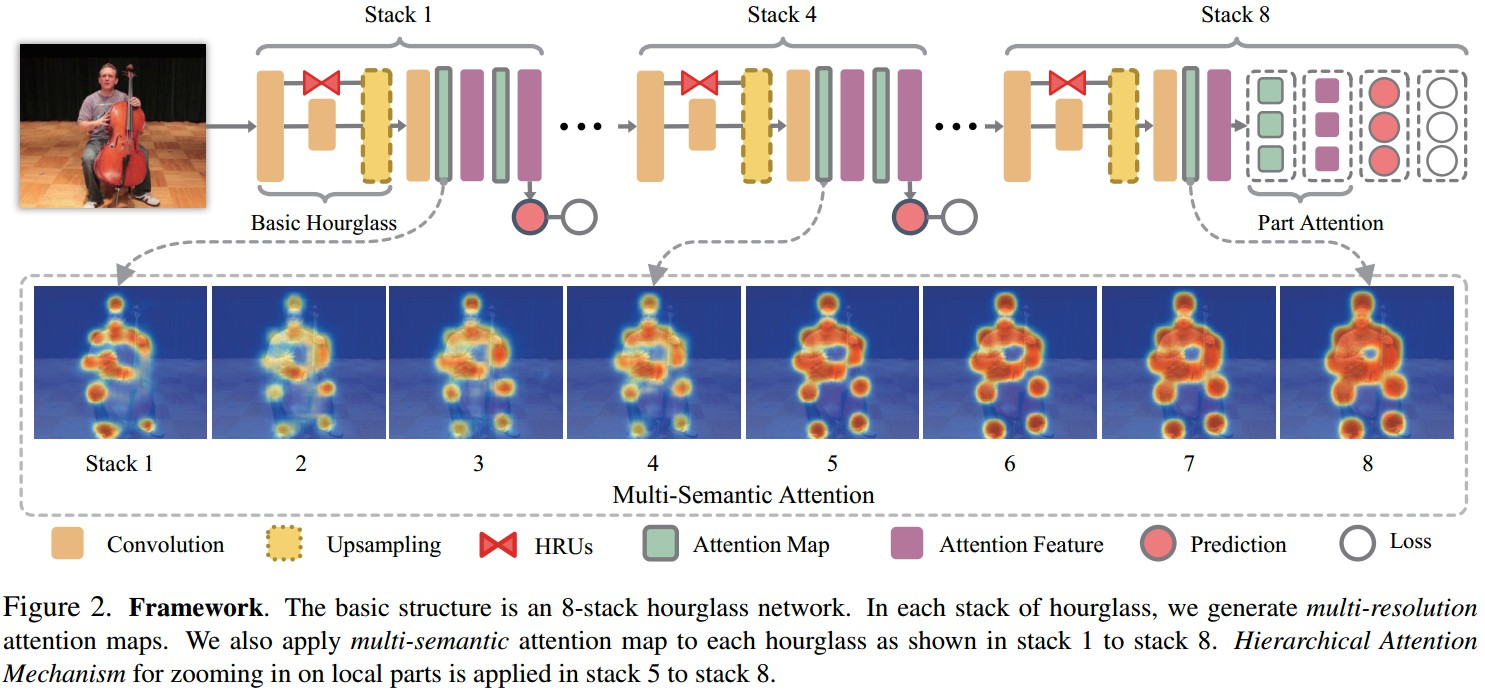Figure2. 8-stack hourglass网络的基本结构. 各hourglass stack 分别得到多分辨率注意力图. 将多语义注意力图应用到各 hourglass，如 stack 1 - stack 8. 分层注意力机制对局部关节点的缩放应用在 stack 5 - stack 8.

### 2.1 基础网络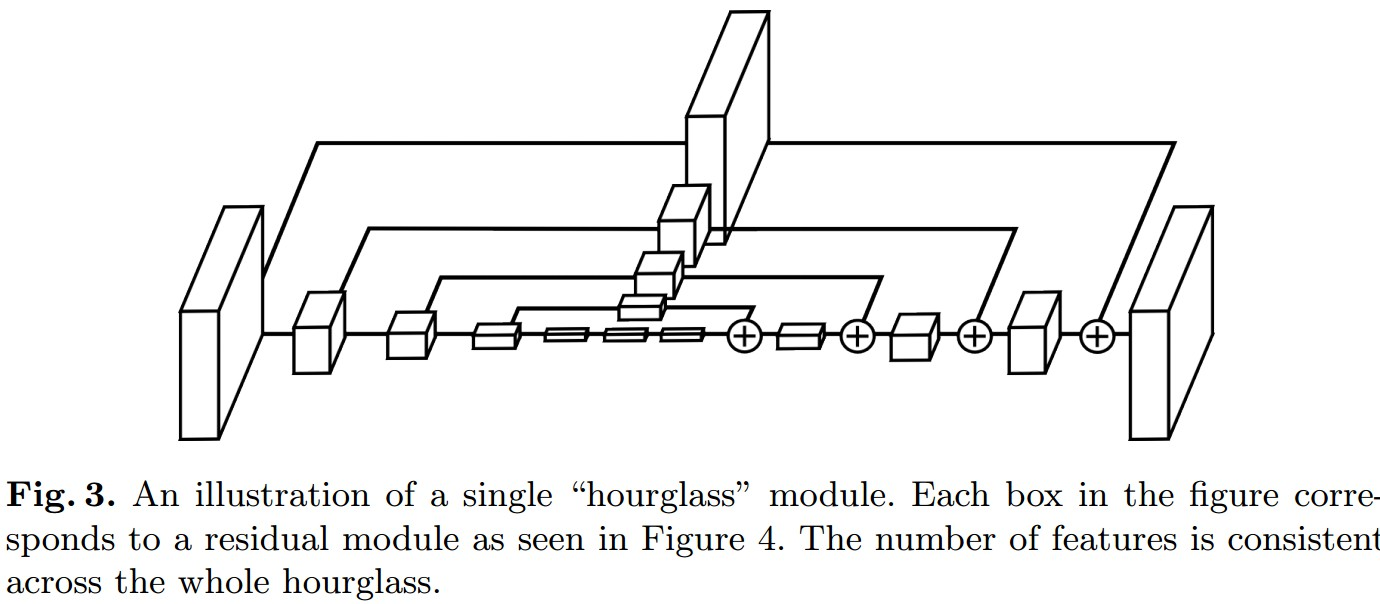### 2.2 Nested Hourglass 网络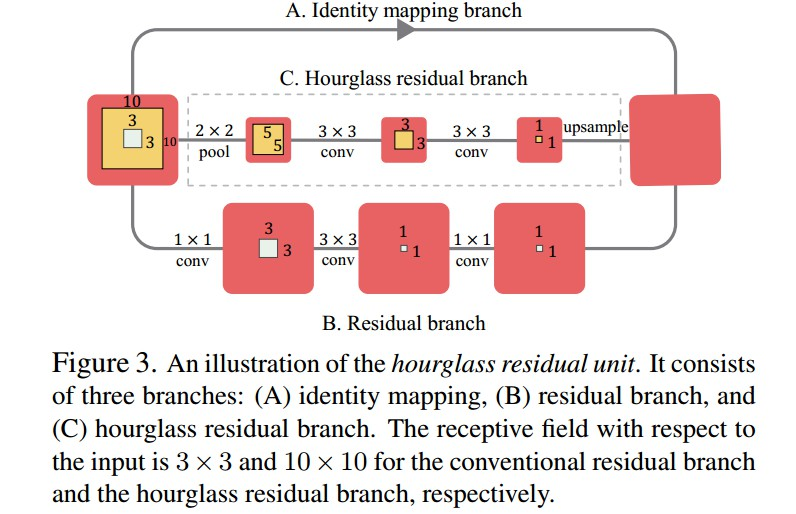Figure3. HRU例示. 包括三个分支：(A)恒等映射(identity mapping)分支； (B)残差分支；(C)hourglass residual 分支. 卷积残差分支和 hourglass residual 分支输入的接受野分别为 $3×3$ 和 $10×10$.

### 2.3 多分辨率注意力(Multi-Resolution Attention)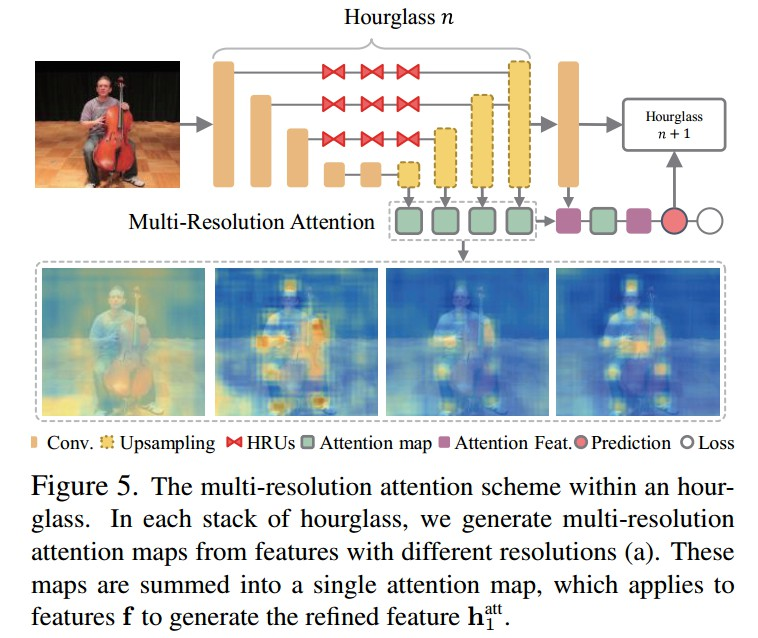Figure5. hourglass 的多分辨率注意力机制.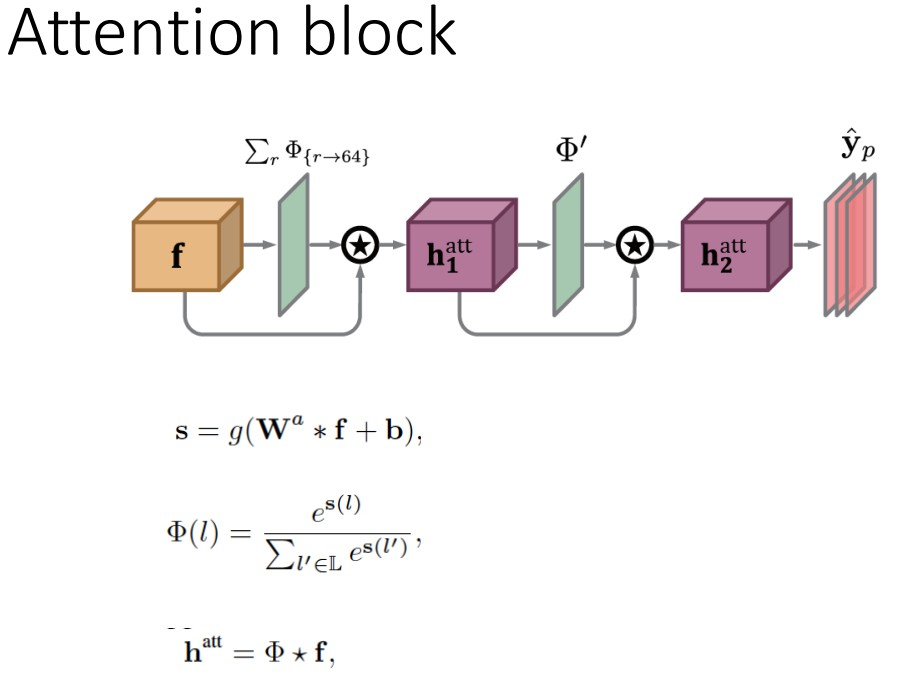Figure4. 注意力机制例示.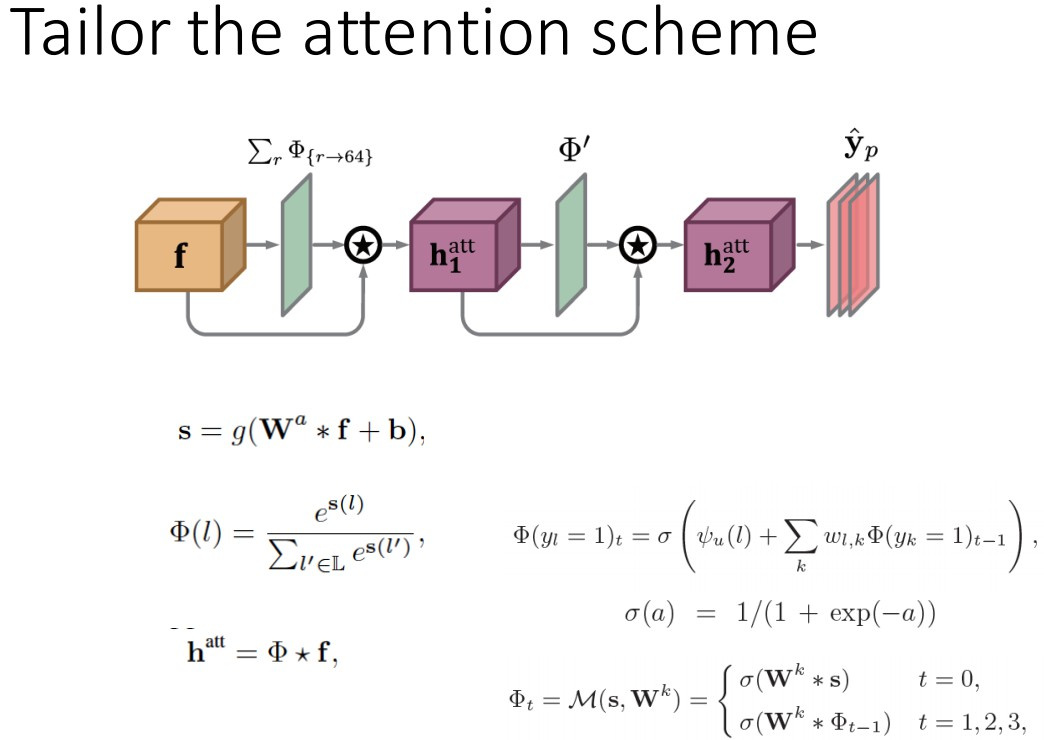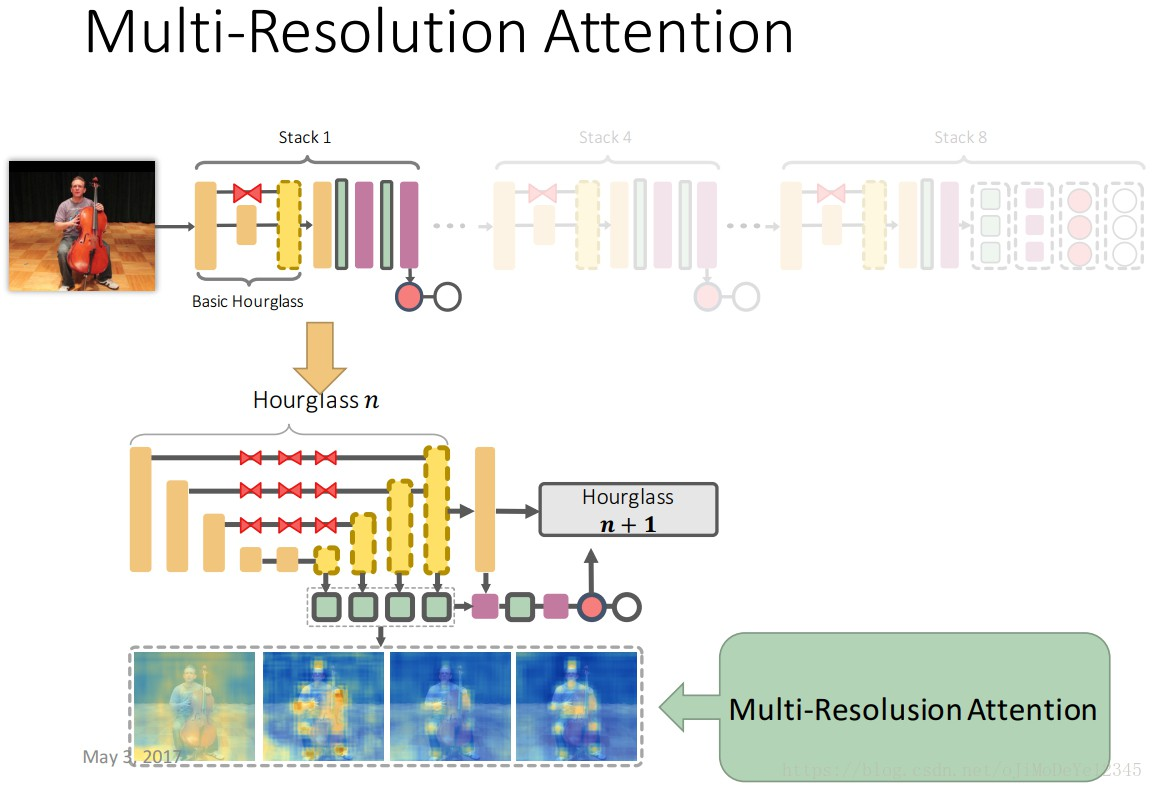### 2.4 多语义注意力(Multi-Semantics Attention )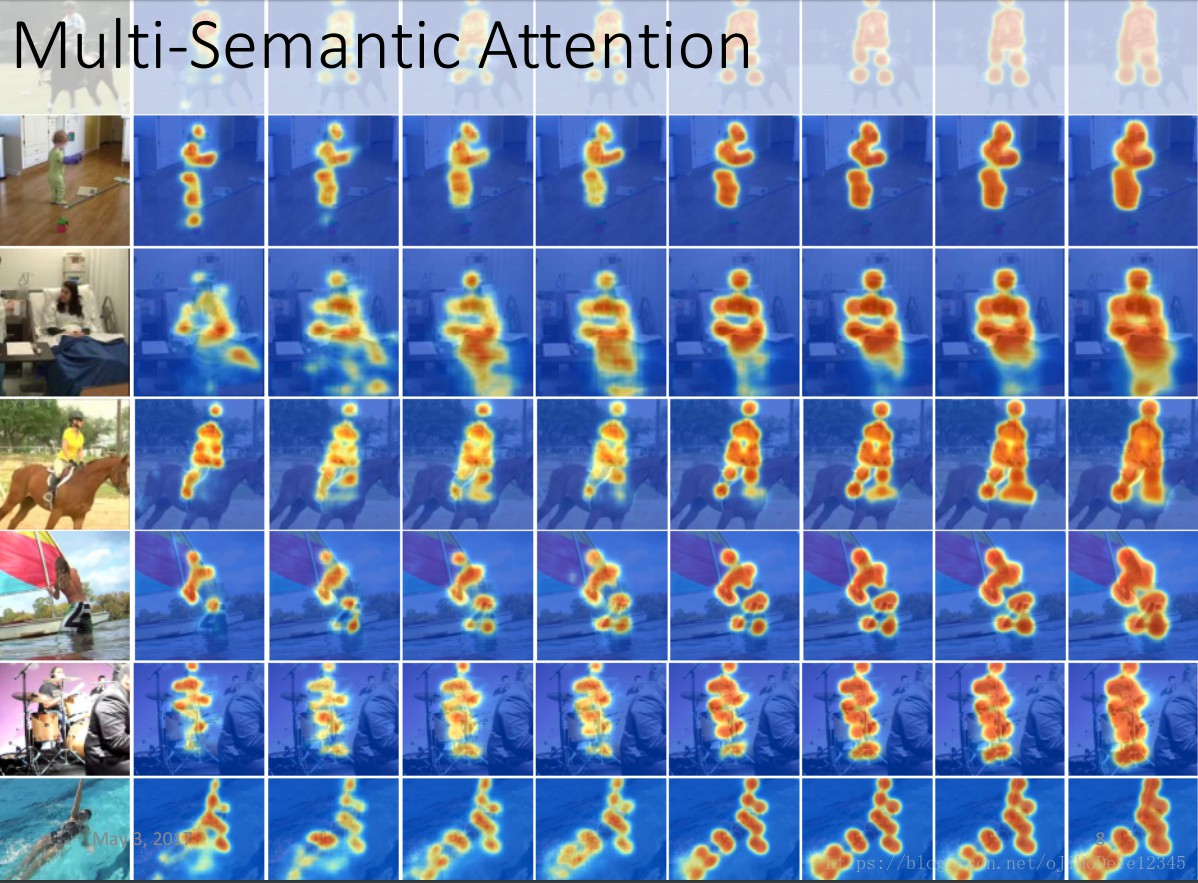### 2.5 分层注意力机制(Hierarchical Attention Mechanism)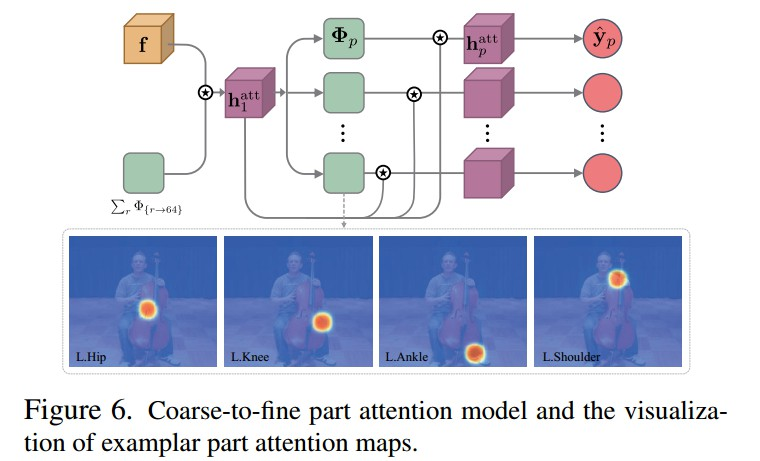${ \mathbf{f} }$ 是Figure5中 hourglass stack 的最后一层的输出特征，${ \Phi }$ 上采样的注意力图.

${ \mathbf{h}_{1}^{att} }$ 是 hourglass stack 输出特征和注意力模型得到的注意力图；

${ \mathbf{h}_{2}^{att} }$ 是注意力图 ${ \mathbf{h}_{1}^{att} }$ 结合各关节点注意力模型得到的refined后注意力图特征.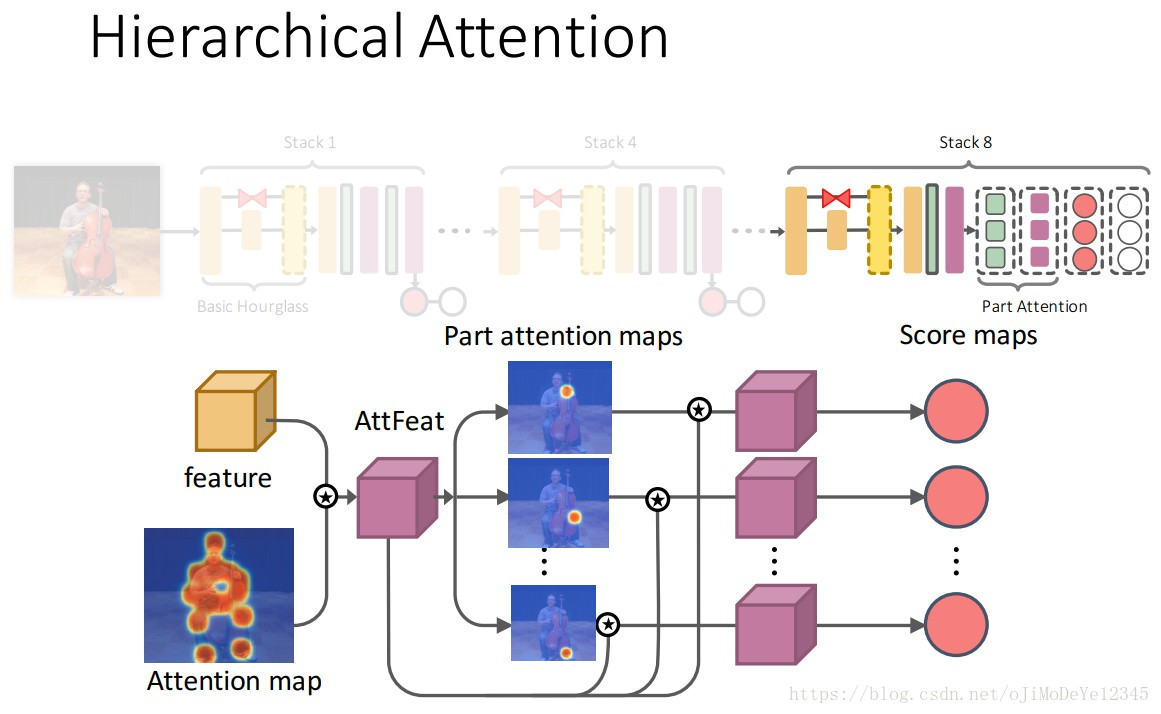### 2.6 模型训练

Hourglass stack 网络估计人体关节点的heatmaps.

${ L = \sum_{p=1}^{P} \sum_{l \in \mathbf{L}} ||\breve{y}_p(l)- y_p(l)||_2^2 }$

${ p }$ 为第 ${ p }$ 个关节点，${ l }$ 为第 ${ l }$ 个位置.

${ \breve{y}_p }$ 为关节点 ${ p }$ 的预测heatmap；

${ y_p }$ 为对应的GT heatmap，是以关节点位置为中心的 2-D Gaussian.

#### 2.6.1 Data Augmentation

• crop the images with the target human centered at the images with roughly the same scale
• 输入图像尺寸 256×256
• randomly rotate (±30◦) and flip the images
• random rescaling (0.75 to 1.25) and color jittering
• Torch7，initial learning rate 2.5 × 10^{-4}，RMSprop 优化.

## 3. Results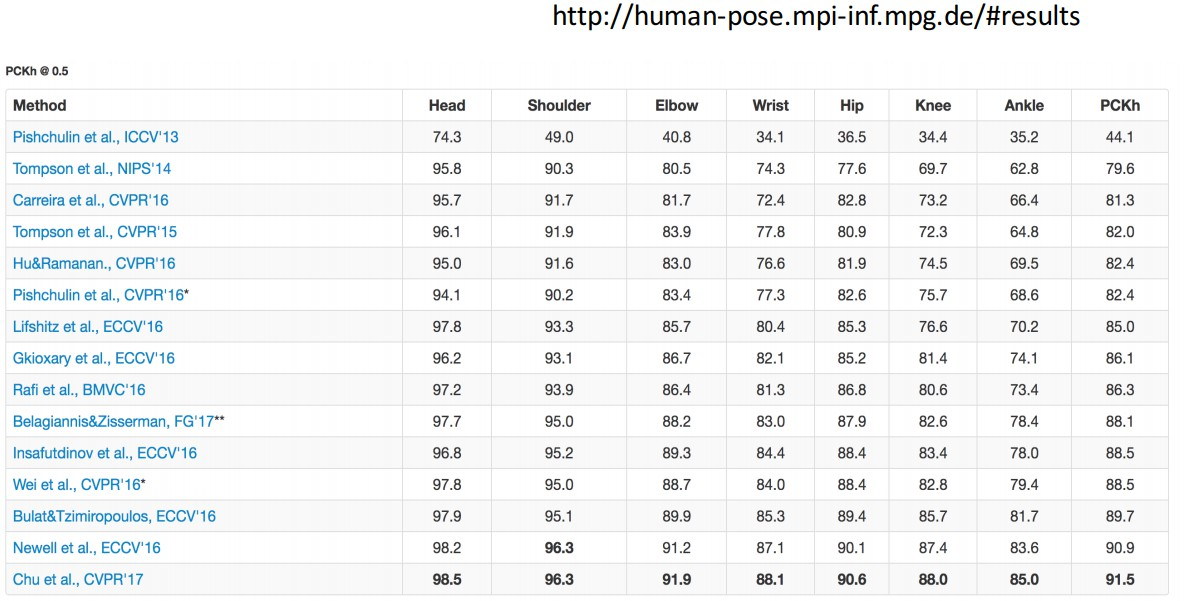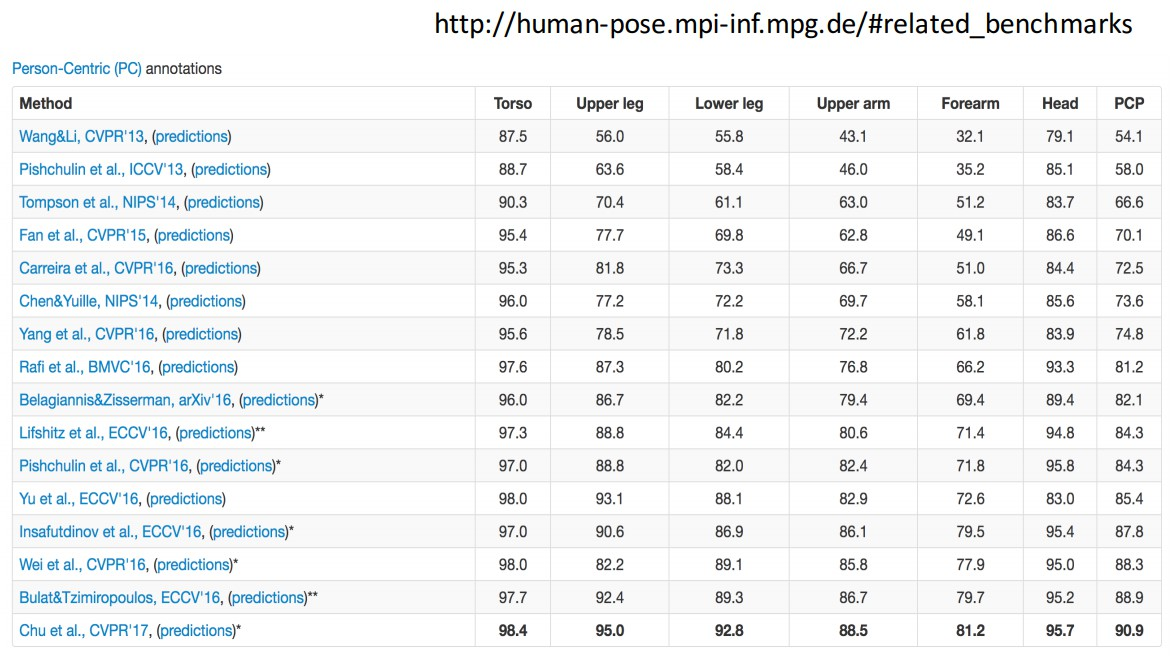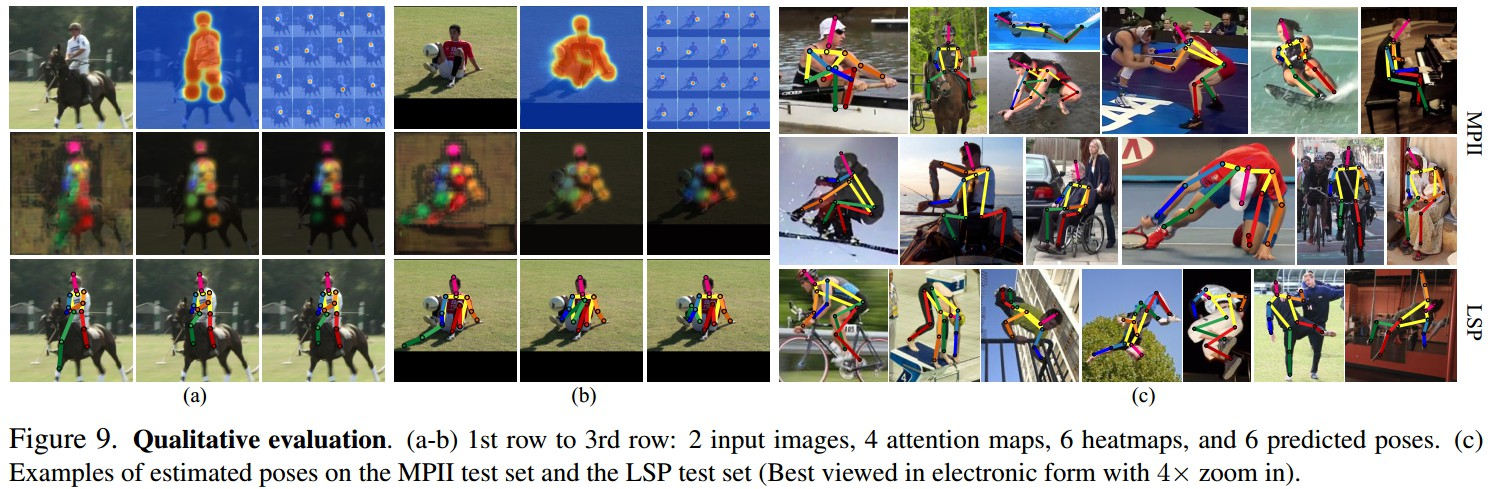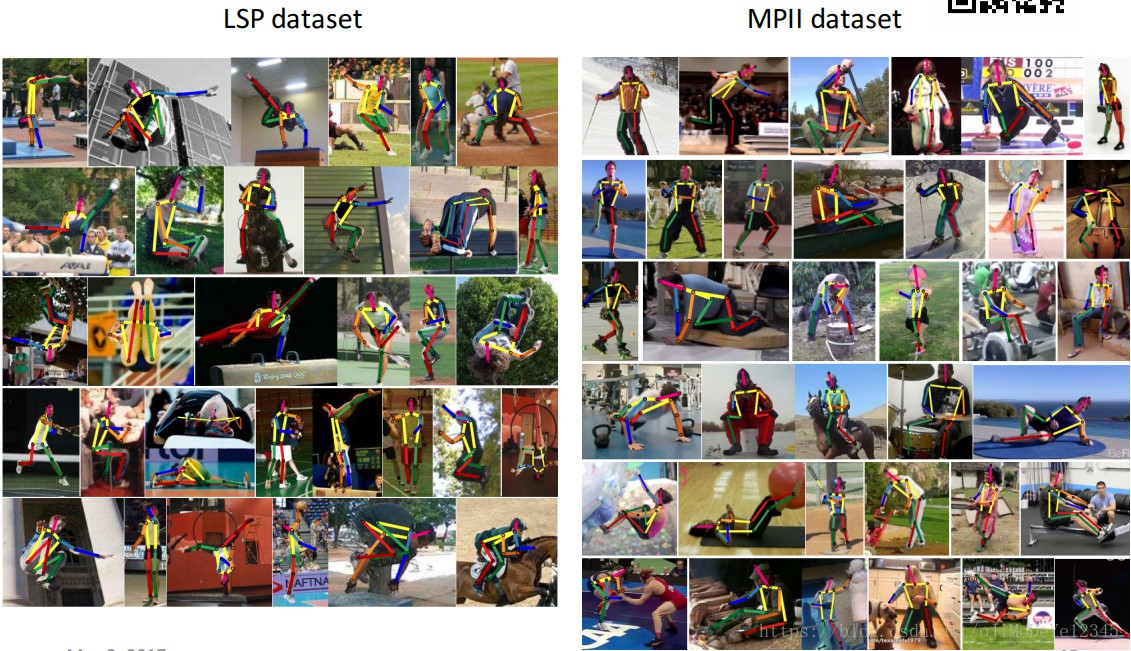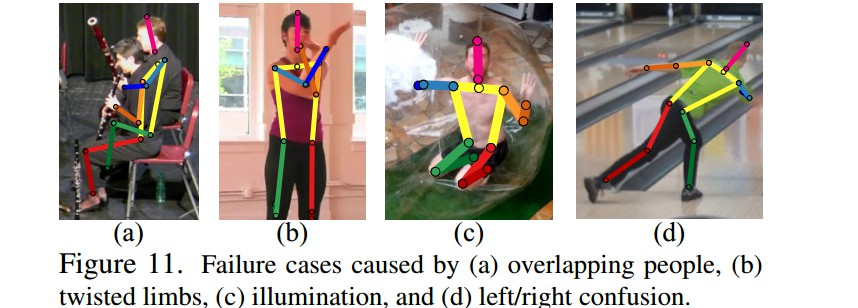Last modification：October 10th, 2018 at 01:08 am